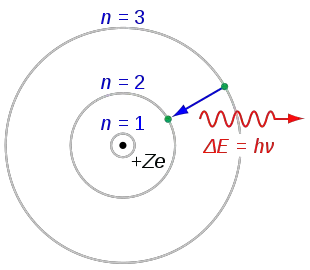# Describe Bohr's Model of the Atom. Drawbacks Ans PostulatesThe Bohr model states that atoms have different electronic configurations in which electrons are revolving around the positively charged nucleus due to electrostatic forces.

After the scientific contributions of John Dalton, Joseph Thomson, and Rutherford's model, Niels Henrik Bohr proposed Bohr's atomic model in 1911.

In other models of atoms, it was stated that charged protons (positively charged particles) were in the nucleus of the atom, and negatively charged electrons circulated in circular orbits around the nucleus. Bohr studied the motion of electrons orbiting the nucleus in atomic theory.

## How Did Niels Bohr Describe Electrons in His Atomic Model?

Niels Bohr describes his model like the Copernicus planetary model, the planets describing circular orbits around the Sun. In this case, the electrons orbit around the nucleus of the atom but only by certain allowed orbits.The orbits are labeled by an integer, the quantum number n.

## What Are the Postulates of Bohr's Model of an Atom?

In 1913, danish physicist Niels Bohr proposed Bohr's theory using the spectral lines of the hydrogen atom and Planck's quantum theory. In light of this information, Bohr's postulates can be summarized as follows:

1. The electrons in an atom move in orbits at a certain distance from the nucleus. Each stable state has constant energy.

2. At any stable energy level, the electron moves in a circular orbit. These orbits are called energy levels or layers.

3. As long as the electron is in one of its stationary states, the atom does not emit light (radiation). However, when it goes from a high energy level to a lower energy level, it emits a light quantum.

4. Stable levels at which electron movement is possible are designated by letters K through Q.

Each orbit has electrons with different levels of energy. The electron jumps from one orbit to another until it reaches one with the appropriate space and level.

The energy level an electron occupies typically is called its ground state. But it can move to a higher-energy, less-stable level or shell by absorbing energy.

The electron, which moves in a circular motion at the energy level closest to the nucleus, is stable. It does not emit light. If the electron has enough energy, it will jump to an energy level higher than the energy level it is at.

According to Bohr's atomic model, the angular momentum of an electron orbiting around the nucleus is quantized.

## What Are the Drawbacks of Bohr's Atomic Model?

• Since electrons are very fast, they must be considered in classical physics and the theory of relativity.

• Bohr's atomic model can only explain the spectra of single electron (hydrogen) atoms. It cannot explain the spectra of multi-electron atoms.

• Bohr's atomic model didn't take into account the Wave-particle duality.

• According to Werner Heisenberg's uncertainty principle, the location and speed of the electron in the atom cannot be determined simultaneously with absolute certainty.

• In this model, he does not mention neutrons.

Author:

Published: April 23, 2021
Last review: April 23, 2021